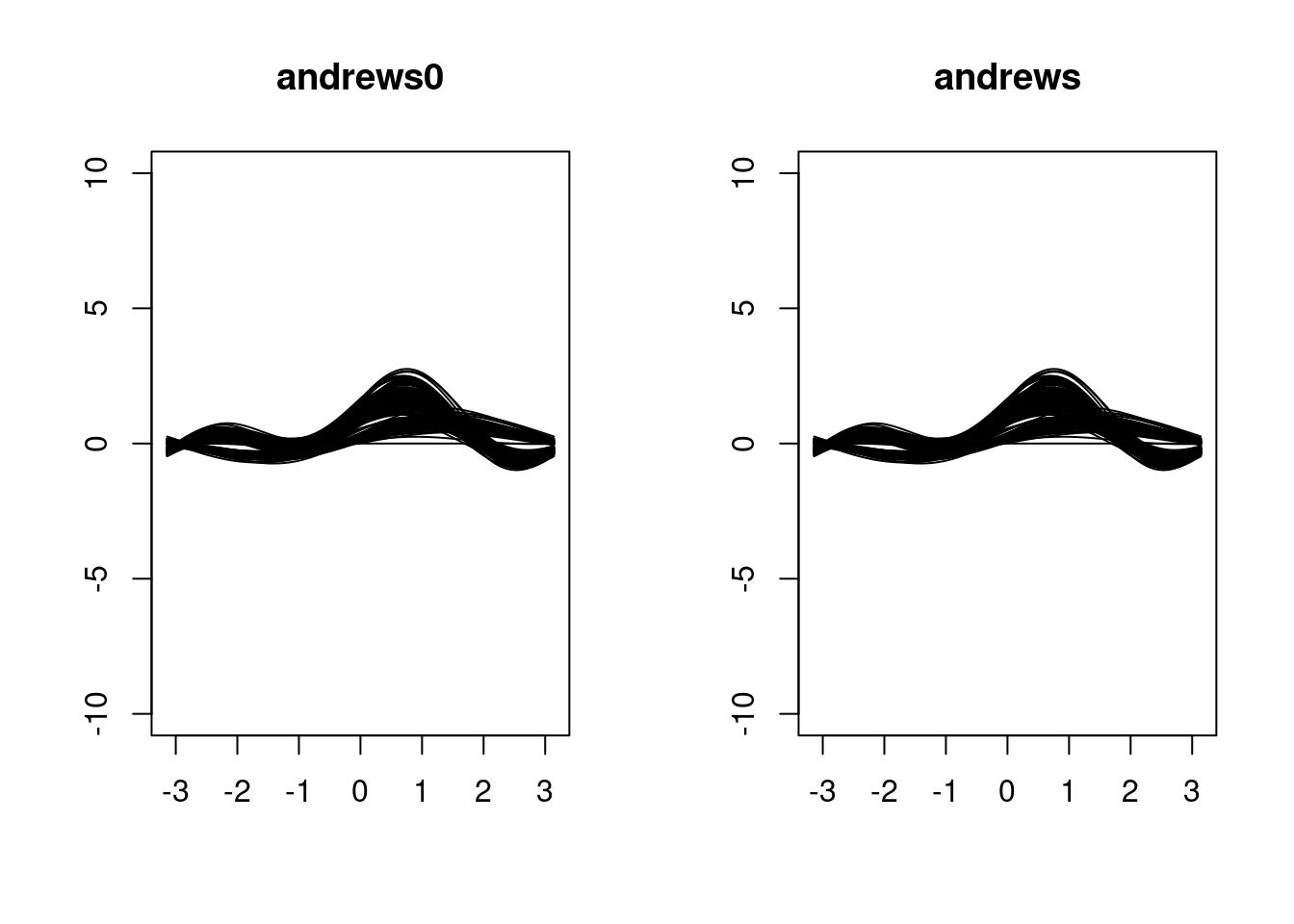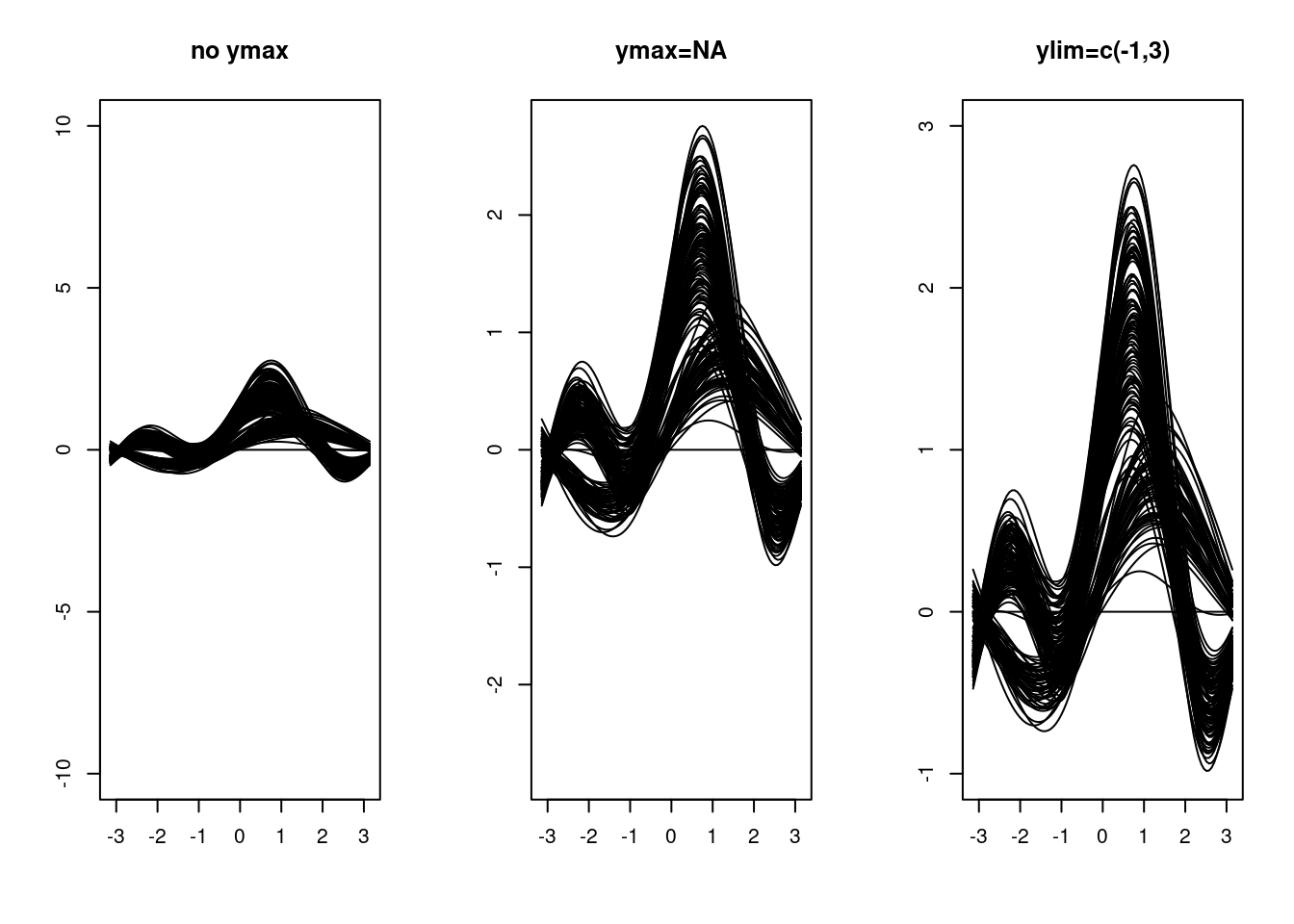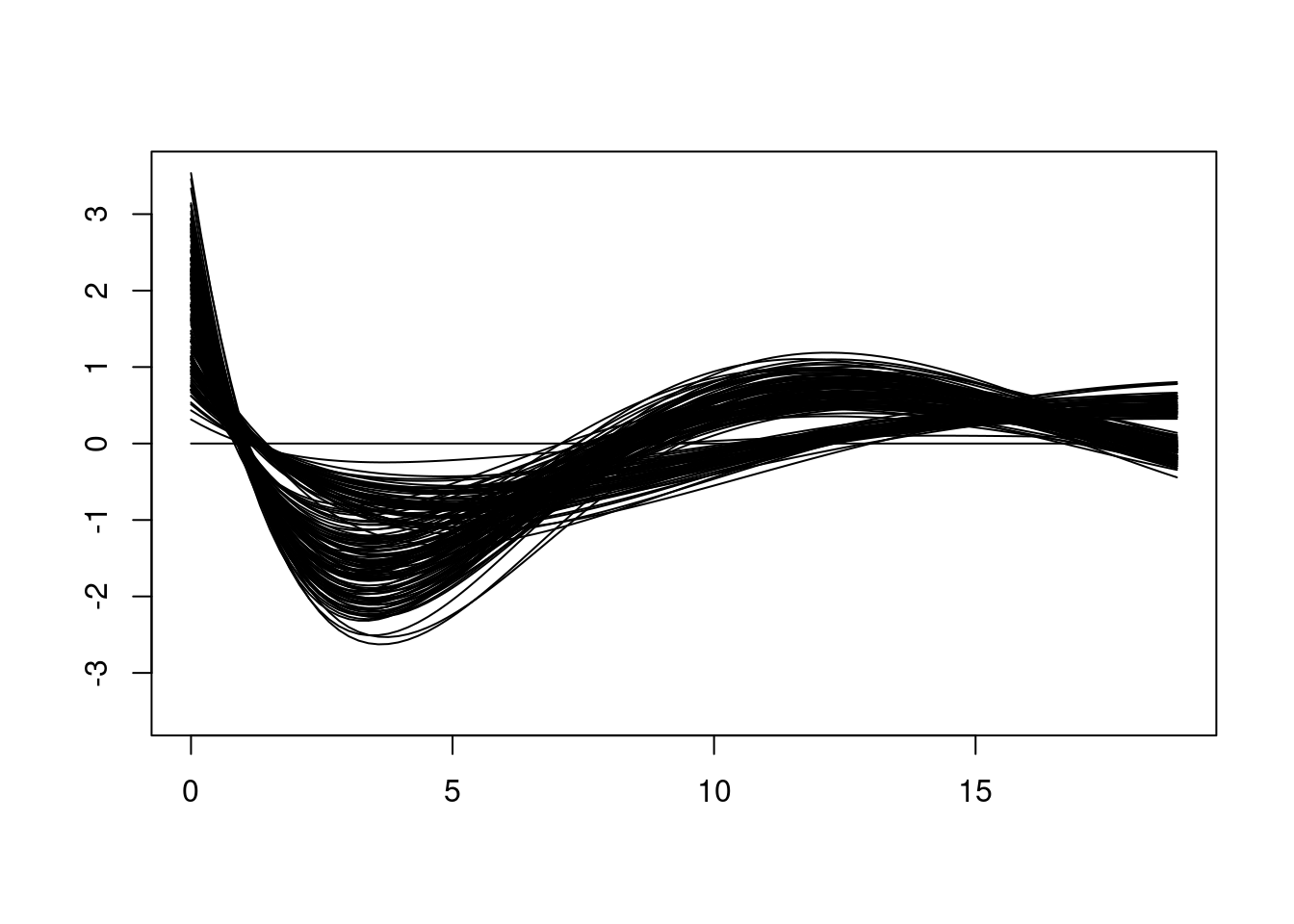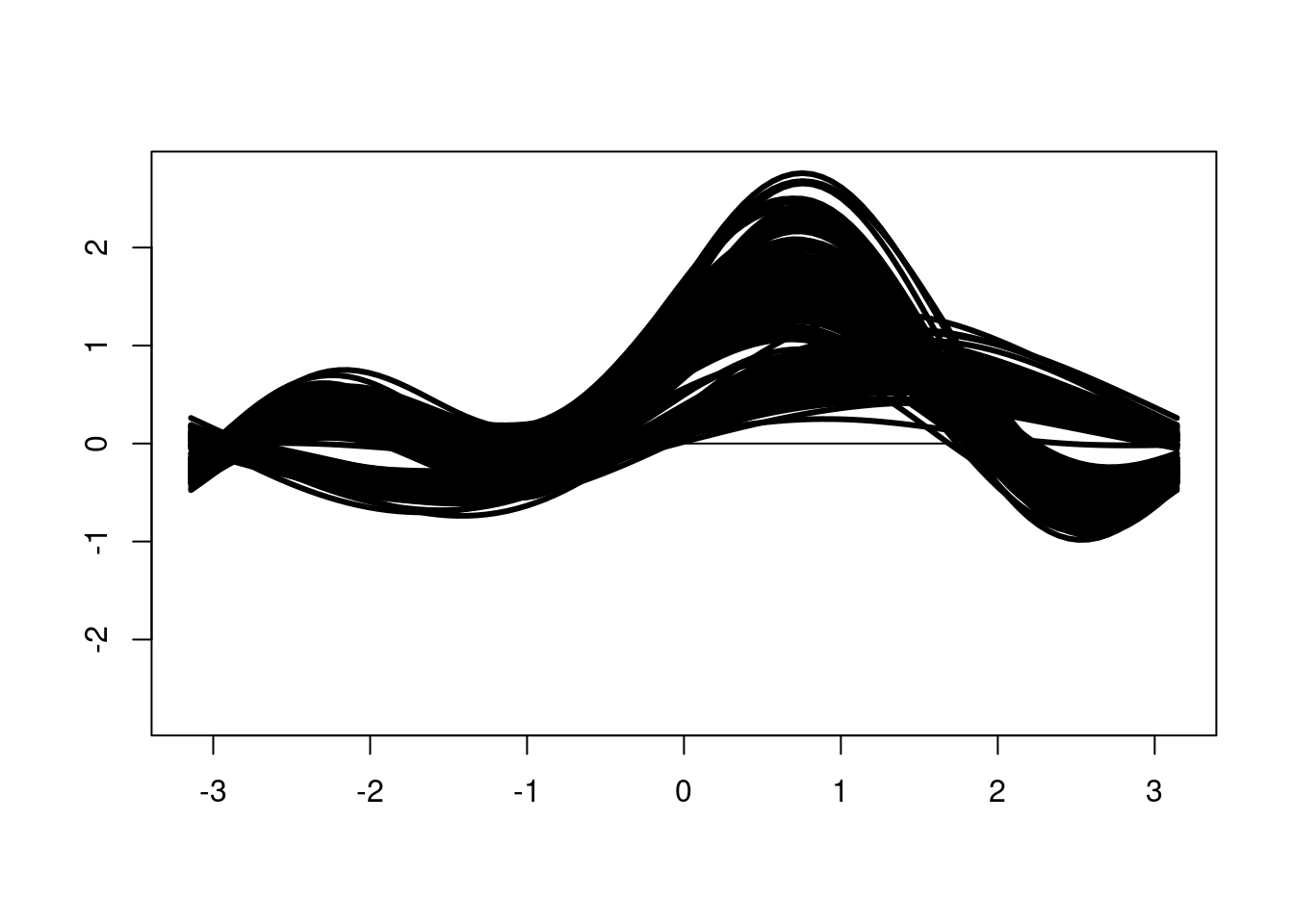``library(andrews)``

# General

The `andrews` routine, implemented by Jaroslav Myslivec, has been rewritten and extended. The old routine is still available as `andrews0` and the rewritten routine should give the same graphics with the same parameter values.

``````op <- par(mfrow=c(1,2))
andrews0(iris, main="andrews0")
andrews(iris, main="andrews")
par(op)``````Some more types of curves has been added. To see more comparisons of `andrews` and `andrews0`, run interactively

``zzz()``

# Extensions

Full parameter list for `andrews` is:

``````andrews (df, type = 1, clr = NULL, step = 100, ymax = 10,          # old parameters
alpha = NULL, palcol = NULL, lwd = 1, lty = "solid", ...) # new parameters``````

The parameters in the call to `andrews` that come in `...` are passed to `plot` to draw the base window:

• The parameters `x`, and `y` cannot be set by the user through `...`.
• Available user parameters are `main`, `sub`, `xlim`, `ylim`, `xlab`, `ylab`, `axes`, `asp` and possibly other parameters.

Note that the setting of `ylim` has priority over the setting of `ymax`.

## Scaling the window height and width

The height of the window can be scaled by setting `ymax` or `ylim`.

1. If `ymax` is not set then `ylim=c(-10,10)` is used.
2. if `ymax` is set to `NA`, then `ylim=c(-lim,lim)`, where the maximum height `lim` is calculated from the curves. Note that this option doubles the calculation time.
3. by setting `ylim` directly.
``````op<-par(mfrow=c(1,3))
andrews(iris, main="no ymax")
andrews(iris, ymax=NA, main="ymax=NA")
andrews(iris, ylim=c(-1,3), main="ylim=c(-1,3)")
par(op)``````The width of the window can be scaled by setting `xlim`, which is particularly useful for `type==3` or `type==5`.

``andrews(iris, type=3, xlim=c(0,6*pi), ymax=NA)``## Line type and width

The parameters `lty` and `lwd` determine the linetype and width. The length of the parameters must be either `1` or `nrow(df)`. In the first case the values are applied to each curve, in the second case `lty[i]` and `lwd[i]` are used for the `i`th line.

``andrews(iris, ymax=NA, lwd=3)``## Colouring the curves

The curves can be coloured individually if `length(clr)==nrow(df)`.

``andrews(iris, ymax=NA, clr=rainbow(nrow(iris)))``